SEARCH HOMEMath Central Quandaries & QueriesQuestion from Sophia, a parent: Susan woke up at 7:42 Am to get ready for school. She slept for 10 and 1/3 hours. What time did she go to bed?Hi Sophia. We can do this question with fractions!

First, let's convert the time to a mixed fraction. There are 60 minutes in an hour, so 7:42 am is: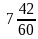Now we need to subtract 10 and 1/3 hours from this.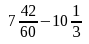But you can see that the number we are subtracting is bigger than the first number, so we will have a negative time! That means the answer is in the previous day, which makes sense when you think about when Susan went to bed. There are 24 hours in a day, so we can add that.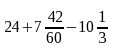To solve this, we need to first change the mixed fractions to improper fractions by absorbing the whole numbers into the numerators: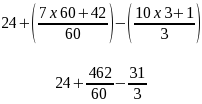Now we need to use a common denominator (60 is a good common denominator for working with time) and then we can solve it to a single fraction: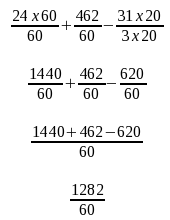This is our answer, but it isn't in a nice form. Let's convert it to a mixed fraction now with hours and minutes.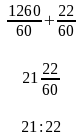Do you know what 21:22 is? It is 21 hours and 22 minutes after midnight. So that means it is 21 - 12 = 9 hours and 22 minutes after noon.

So the result is 9:22 pm.

Hope this helps!
Stephen La Rocque.Math Central is supported by the University of Regina and The Pacific Institute for the Mathematical Sciences.